# Enhancement of Gain and Reduction of Backward Radiation Using Metasurface Antenna for Energy Harvesting Applications

Enhancement of Gain and Reduction of Backward Radiation Using Metasurface Antenna for Energy Harvesting Applications
Dept of ECE, CCET Degree Wings Chandigarh Panjab University, Chandigarh 160019, India

Dept of ECE, Sri Vasavi Engineering College, JNTUK, Andhra Pradesh 534101, India

Corresponding Author Email:
mullangi_ece@ccet.ac.in
Page:
755-762
|
DOI:
https://doi.org/10.18280/ts.390241
15 February 2022
|
Accepted:
6 April 2022
|
Published:
30 April 2022
| Citation

OPEN ACCESS

Abstract:

A reflective metasurface technique is used to enhance the gain and reduction of backward radiation of an antenna. A normal metal slots is placed around the radiating patch to get the improved gain. Another metasurface antenna is placed with 10mm Gap from the radiating antenna to get the high radiation and enhanced directivity. A partial ground and line feeding with the conventional coplanar wave guide technique is used to improve the over all result. A rectifier circuit has been designed to harvest the electromagnetic energy to convert the DC output power. In the proposed model 8.1dB of Gain and -13.1dB of backward radiation with 2.82V of DC output power has been observed. The entire simulation and measurements has been done on HFSS and ADS software.

Keywords:

reflective metasurface, rectifier, conventional CPW, electromagnetic energy

1. Introduction

Metasurface is one of the important techniques in the 5G technology to get the enhanced results with the miniaturized antenna . By using the metasurface antennas a more RF energy can be harvested . Generally metasurface antenna has a left handed properties which has both permittivity and permeability are negative with negative refractive index .

Rectenna (Rectifier + filter) is a significant technology for collecting electromagnetic energy from the receiving antenna . The Impedance matching network, Rectifier circuit, and Voltage doubler circuit make up the Rectenna .

Over the past few years a lot of research has been going on in the field of wireless power transmission. Liu et al. designed a rectangular patch with the dual substrate and successfully achieved the 3.57 dBi of forward gain and 7.5 dBi of backward Gain . Zhao et al. had designed the 4x4 complementary split ring resonator with the 74cmx74cmx1mm and achieved very low efficiency with the 35.1% of 10dbm of input power .

Behera et al. designed a partial ground metasurface aerial and obtained the 950MHz of the axial ratio Bandwidth with the 85% transmission efficiency . El Badawe et al. have successfully achieved the 40% Ac to DC power conversion . Aldhaeebi and Almoneef had proposed an antenna with the dual polarization with the 98% of AC to Dc power conversion efficiency .

Hu et al. has achieved the 25MHz of bandwidth at 2.45GHz of resonating frequency . Zhang et al. had designed the stair case rectangular shape slots on the radiating patch and achieved the high of 4.5dBi of gain with the low bandwidth and low output power . Ghaderi et al. simulated the rectangular slot with the 9x9 phased array and they found the very low bandwidth 100MHz at 2.4GHZ resonating frequency .

From the (Table 1) Literature review it was observed that the Most of the metasurface antenna with RF energy harvesting applications has achieved low bandwidth and low Gain. This paper aims to design the metasurface antenna with the high Gain and high bandwidth to achieve the high output Voltage. Liu et al. 2019  achieved the Gain of the designed antenna is 3.5dB and 12.8dB of the Backward Radiation. For the proposed antenna it was founded the Gain of the Antenna is 8.0 dBi and Backward Radiation is -13.1dB. In the proposed antenna the Receiving the antenna Efficiency is 93.5% with 113.5 of Front to back ratio.

Table 1. Literature review

 Ref Shape of the patch antenna Bandwidth Gain Advantages 6 Rectangular patch with rectangular slots on the Ground plane 110MHz 3.57dBi Achieved Low backward radiation 7 4x4 complementary split-ring resonator (CSRR) array - - 35.1% of efficiency had achieved at -10dB input power 8 Schematic diagram of proposed MTS with partialground antenna 5MHz 3.4dBi Axial ratio bandwidth is 950MHZ 9 Plus shaped 8x8 array antenna with the dual substrate Measured efficiency AC to DC conversion is 40% 10 Geometry of dual polarization of proposed MTS antenna 1.7GHz - Achieved the higher radiation efficiency 98% 11 MTS for unit cell with gap on Closed rectangular patch 25MHz - Efficiency was achieved for 2mm and 6mm air gap 12 Stair case spiral shape patch with CPW technique 25MHz 4.45dBi Half power beam width =68.30 13 Rectangular slot with plus shaped 9x9 array antenna 100MHz Achieved 90% of efficiency higher for every polarization angle

In the section II antenna design is discussed with dimension and results has discussed in the section III whereas Rectifier circuit had discussed in the Section IV and the fabricated model presented in the Section V followed by the comparison table.

Table 1 shows the literature review of the metasurface antenna for RF energy harvesting applications. This paper focuses on design the metasurface antenna with High Gain and improve Bandwidth to achieve the high output Voltage.

In open literature, a good receiving antenna efficiency achieved by using reflector but not gain and bandwidth [7, 9-11, 13].

2. Antenna Design

Metamaterial is the three dimensional in structure with the artificial medium, due to its negative permittivity and permittivity the Gain or radiation efficiency improved with the less dimensions of the antenna. The main drawback on metamaterial is manufacturing very difficult in the bulk quantity . Metasurface is the two dimensional in the structure with the negative permittivity and permeability, due to its 2D structure it is very easy for manufacturing. So it is one of the booming technology in the present research .

As one of the most suitable antenna for the receiving the electromagnetic energy is the metasurface antenna. The proposed antenna has been designed with the dimensions 96mmx84mmx1mm of each substrate with the FR4 is the dielectric substrate.

A rectangular slot is present around the radiating patch antenna on the top substrate, and a 4x4 square form Defective ground shape is present on the reverse side. The FR4 substrate has a reflective metasurface with dimensions of 96mmx84mmx1mm, and the gap between the two substrates is 10mm. The backward radiation is eliminated by using a reflecting metasurface, which improves the antenna's gain and backward radiation.

Due to the Reflective metasurface the Backward radiation is reduced, so the receiving energy from the antenna is also improved. In this paper the Rectenna is designed with LC-impedance matching network with the Voltage Multiplier Circuit and achieved the 2.82V of DC voltage and 112µA of DC current.

The Figure 1 indicates the Geometrical view of the proposed antenna with the front view of the proposed aerial, Figure 2 shows the back view of the substrate 1. The Figure 3 shows the front view of the metasurface reflector. Figure 4 represents the back view of the metasurface reflector with the defective ground structure.

## 1.pngFigure 1. The characteristics of top view of proposed antenna

## 2.png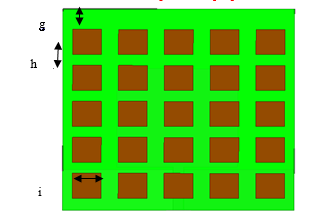Figure 2. The characteristics of back view of the top substrate (back view)

## 3.png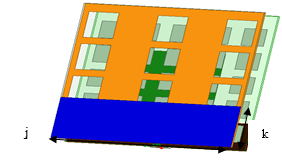Figure 3. The characteristics of proposed aerial with the partial ground (back view)

## 4.png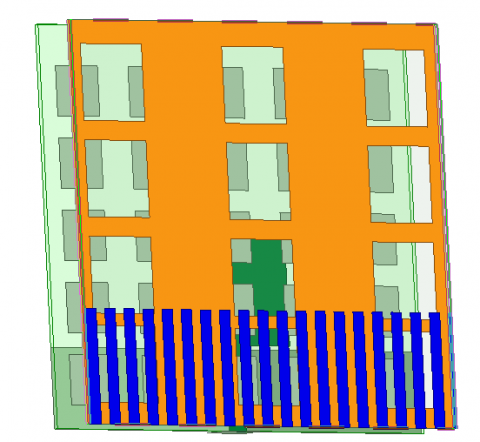Figure 4. The characteristics of the proposed antenna with DGS (back view)

Table 2. Dimensions of the proposed aerial

 Letter Parameter Dimension a Width of the Patch 0.4 $\lambda_{0}$ b Length of the Patch 0.328 $\lambda_{0}$ C Width of the Substrate 0.768 $\lambda_{0}$ d Length of the Substrate 0.672 $\lambda_{0}$ e Length of the Ground on the top substrate 0.136 $\lambda_{0}$ f Width of the Ground on the top substrate 0.3656 $\lambda_{0}$ g The Gap of the slot on the back side of the Top substrate 0.065 $\lambda_{0}$ H Length of the slot on back side of the Top substrate 0.084 $\lambda_{0}$ i Width of the slot on back side of the Top substrate 0.096 $\lambda_{0}$ j Width of the Partial Ground 0.768 $\lambda_{0}$ K Length of the Partial Ground 0.201 $\lambda_{0}$

The Table 2 shows the dimensions of the suggested antenna and it is mesured using the FR4 dielectric substrate. The size of suggested aerial is 0.768λ0×0.672λ0×0.008λ0 with the gap between two substrates are 10mm.

3. Results and Discussions

In this section various aerial parameters can be discussed with the simulated and measured results.

A. Return loss

Return loss can be defined as the how much power is reflected back from the input terminal. Reflection coefficient is defined as .

$\Gamma=\frac{v_{r e f}}{v_{f w d}}$       (1)

where, $\Gamma$ is reflection coefficient.

For the designed antenna the reflected power is 52mW and the radiated power is 910.05mW. So the reflection coefficient is 58.31mW.

Return loss is calculated from

RL = -20log (ᴦ) = -24.68dB       (2)

From the Eq. (2) the return loss is calculated as -24.68dB, whereas the by using the software it was founded for the designed aerial has been operated in dual band and the return loss is -24 dB at 2.4GHz frequency. It was observed that the 480MHZ and 770MHz of bandwidths at 2.4GHz, 6GHz resonating frequency.

## 5.png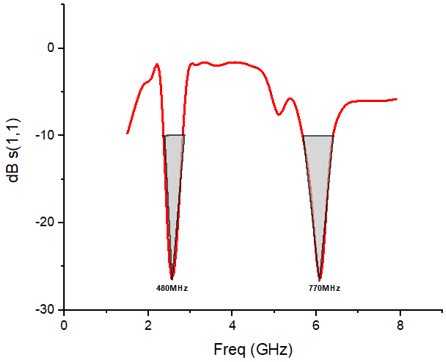Figure 5. Return loss of the designed aerial

In the Figure 5 the shaded region shows the bandwidth of 480MHZ for the designed aerial.

B. VSWR

VSWR is termed as voltage standing wave ratio. It is calculated by the Eq. (3)

$v s w r \approx \frac{1-|\Gamma|}{1+|\Gamma|}$       (3)

where, $\Gamma$ is reflection coefficient.

It was observed that the simulated VSWR for the designed aerial is 1.02. The Figure 6 shows the VSWR of the designed aerial.

C. Gain

In general gain is calculated by using the comparison method. Here the comparison can be done with the designed antenna with either diploe aerial or half dipole aerial or Isotropic antenna.

Directivity for the rectangular patch can be given by the Eq. (4) .

$D=\frac{4\left(k_{0} w\right)}{\Pi \eta G_{r a d}}$        (4)

where Grad is radiation conduction of the patch, $\eta$ is efficiency, k0 planks constant.

In general gain cannot be less than directivity because the efficiency is in between 0 and 1.

$\mathrm{G}=\eta \mathrm{D}$      (5)

where, G is gain of antenna, $\eta$ is efficiency, D is directivity of antenna.

It was founded that the gain for the designed aerial is 8.0dB and it is shown in the Figure 7.

## 6.pngFigure 6. VSWR of the designed aerial

## 7.png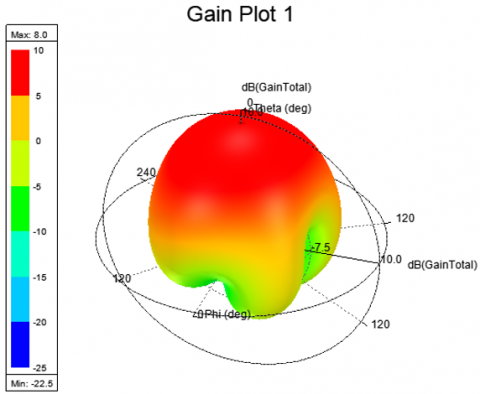Figure 7. Gain plot of the designed aerial

D. Current distribution

The electric field of the radiating patch can be shown in the Figure 8 and the maximum electric filed that can be represents in the red color. The Figure 8 shows the E-plane of the designed aerial and Figure 9 shows the H-plane of the designed aerial.

The magnetic field distribution on the radiating patch is given in the Figure 9 and the maximum magnetic field is 5.5A/m.

The maximum electric field for the designed antenna is 2019V/m and the maximum Magnetic field is 5.5A/m.

## 8.png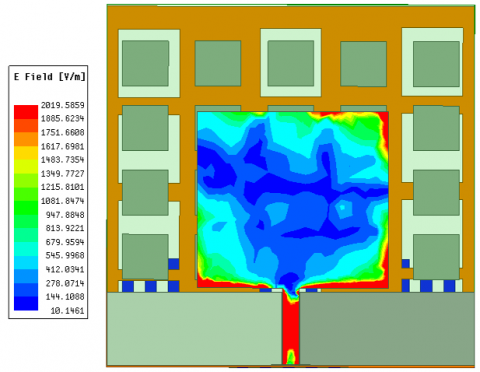Figure 8. Electric field (E-plane) distribution of the designed aerial

## 9.png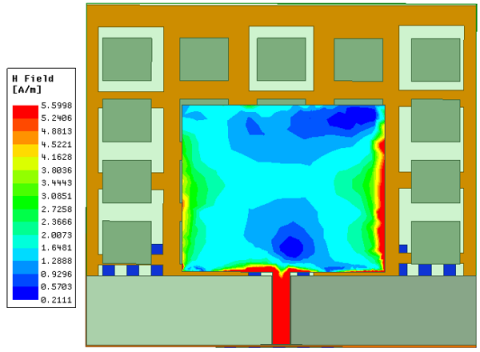Figure 9. Magnetic field (H-plane) distribution of the designed aerial

Radiation pattern is defined as the “Mathematical representation of the radiation properties of an aerial” .

In general Radiation pattern is calculated by using the Eq. (6)

$P_{r a d}=\frac{1}{2} \operatorname{Re} \iint_{S} E_{1} \mathrm{XH}_{2}^{*} d s$       (6)

$=\frac{1}{2 \eta} \iint\left(\left|E_{\theta}\right|^{2} \mathrm{X}\left|E_{\theta}^{X}\right|^{2}\right) r^{2} \sin \theta d \theta d \phi$              (7)

The Eq. (7) shows the Radiation pattern of the Microstrip patch antenna. where electric field E within the patch is normal to the patch and the Ground and Magnetic field is parallel to the patch.

The Figure 10 and Figure 11 indicates E-plane and H-plane of the radiated metasurface antenna.

Backward radiation was -12dB with a gain of 3.5dB and a 10mm gap between the two substrates in the previous design . However, with a 10mm of air gap between the two substrates, the suggested antenna's backward radiation is measured at -12.9dB.

## 10.png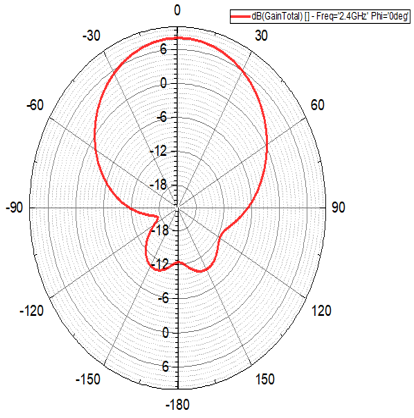Figure 10. E-plane of the designed antenna at 2.4GHz

## 11.png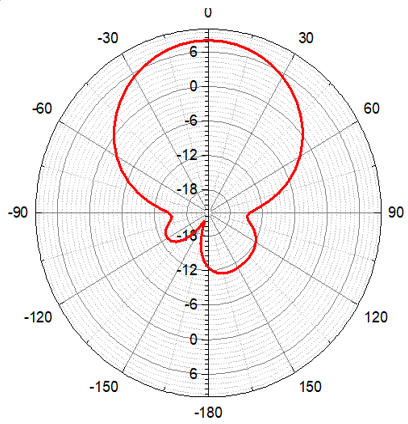Figure 11. H-plane of the designed aerial at 2.4GHz

## 12.png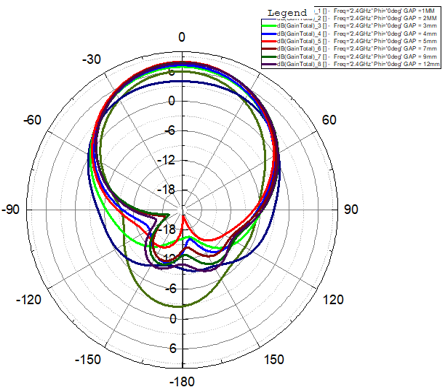Figure 12. E-plane of the designed antenna by varying the Gap with the DGS at 2.4GHz

## 13.png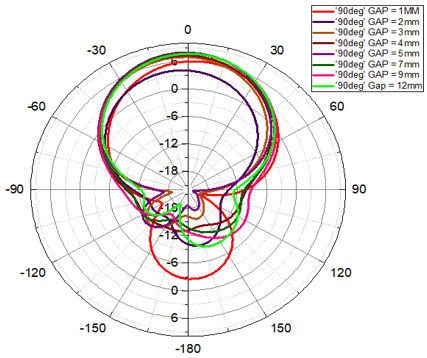Figure 13. H-plane of the designed aerial by varying the gap with DGS at 2.4GHz

## 14.png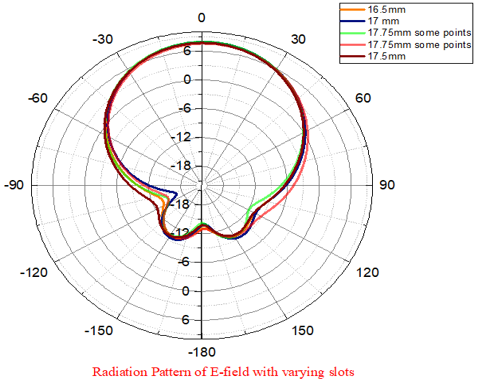Figure 14. Radiation pattern of E-plane with varying the slots at 2.4GHz

F. Parametric analysis

The gap between the two substrates in the proposed aerial varied from 1mm to 12mm. The E-plane of the planned antenna is shown in Figure 12 and H-plane is shown in the Figure 13.

The backward radiation for the designed aerial is -12.9dB, although the Gain and radiation both modified by altering the distance. Figure 12 and 13 depict the aerial's radiation pattern as the gap for the E-plane and H-plane is varied. When the gap between the two substrates is small, the Backward radiation is large, and vice versa, implying that the gap is inversely proportional to the Backward radiation. As compared to previous designs, the backward radiation is very low after adjusting the gap at 10mm and 12mm.

It was observed that by varying the slots width on the backside of the top substrate the radiation pattern can be vary which is shown in the Figure 14 and Figure 15.

G. Efficiency

In general efficiency is defined as the power radiated by the Microstrip patch antenna to the receive power .

$D=\frac{\text { Radiated power }}{\text { Received power }}$

For the designed antenna the efficiency is 93.35% with the front to back ration is 113.8.

## 15.png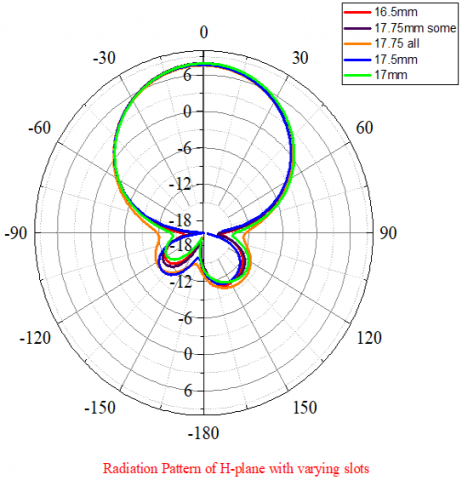Figure 15. Radiation pattern of H-plane with varying the slots at 2.4GHz

4. RF Energy Harvesting

Rectenna is one of the important techniques to harvest more electromagnetic energy from the receiving antenna. The Figure 16 shows the block diagram of the RF Energy harvesting.

## 16.png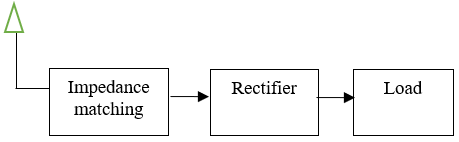Figure 16. Block diagram of the RF energy harvesting system

## 17.pngFigure 17. Rectifier circuit o the designed antenna

The block diagram of the RF energy collecting system is shown in Figure 16. The signal is received by the antenna once it has been transmitted and fed into the Impedance matching network. After matching the impedance, the signal is delivered into the Rectifier, which transforms the alternating current to direct current. It can then be transmitted into a Doubler circuit or a DC-DC Voltage Booster circuit to acquire the highest voltage, which can subsequently be supplied to the load.

For the designed aerial to receive signal from the receiving antenna, impedance matching network can be designed by using the TLIN (lambda/4 transmission line) with the LC matching network. The inductance and capacitance value is calculated by Eq. (8)

$f=\frac{1}{2 \Pi \sqrt{L C}}$       (8)

From the Eq. (8), by taking the frequency as 2.4GHz then the inductance as 525nH and capacitance as 0.02275pf.

The Figure 17 shows the Rectenna designed using the HSMS2860 diodes. Two diodes are connected in forward and backward directions with two capacitors (c1= 541mf, c2 = 600mf) in the suggested circuit.

A. Return loss

From the reception of a signal from the receiving aerial. The signal is routed to the impedance matching network, which should match the desired frequency with the received signal. To determine whether the received signal is correctly aligned with the receiving aerial, the S11 parameter should be aligned with the receiving aerial which is shows in the Figure 18.

The Figure 18 shows the S11 parameter for the designed Rectenna. In the earlier harvesting circuit the maximum output voltage is 1.982V. In the designed circuit the output voltage is 2.82V which is shown in the Figure 19.

## 18.pngFigure 18. Return loss of the designed rectifier circuit output voltage

## 19.pngFigure 19. Output voltage for the designed circuit

## 20.pngFigure 20. Output current of the designed circuit

The Figure 19 shows the output voltage of the Rectifier circuit is 2.82Volts and Figure 20 shows the output current of 110.58μf for the designed rectifier circuit.

5. Fabricated Antenna

The Figure 21 shows the fabricated model of the proposed antenna. The designed antenna has fabricated on FR4 substrates.

## 21.png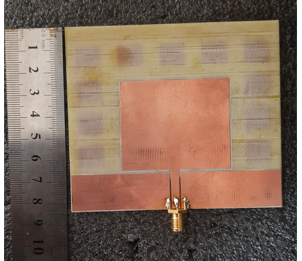Figure 21. Fabricated antenna on top view

## 22.png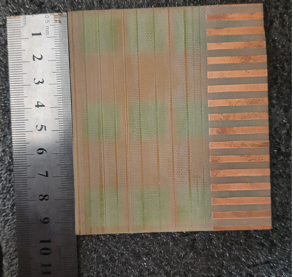Figure 22. Fabricated antenna on back view

## 23.pngFigure 23. Fabricated antenna on front view of the bottom substrate

## 24.png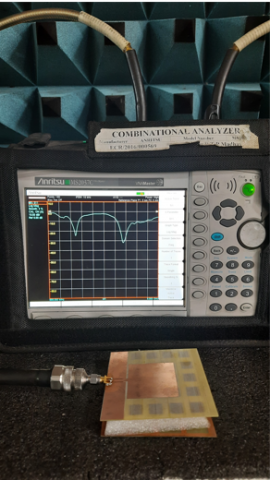Figure 24. Measurement of the S11 parameter using network analyzer

## 25.pngFigure 25. S11 parameter of the measured antenna

## 26.pngFigure 26. VSWR parameter of the measured antenna

Figure 22 and Figure 23 show the fabricated model of the back view & front view of the top substrate. Figure 24 and Figure 25 show the measuring of the S11 parameter using the network analyzer. Figure 26 shows the VSWR measurement for the suggested aerial. It has been observed that the fabricated model exhbits the dual band frequency. Figure 27 and Figure 28 show the measured and simulated gain at 2.4GHz and 6GHz respectively.

## 27.pngFigure 27. Radiation pattern of the designed antenna at 2.4GHz

## 28.png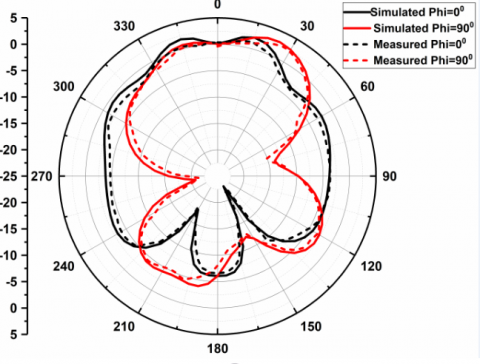Figure 28. Radiation pattern of the designed antenna at 6GHz

6. Comparison

The Table 3 shows the comparisons of the proposed work with the existed literature.

Table 3. Comparison of the proposed antenna with the existed work

Ref No

Liu et al. 

Zhao et al. 

Behera et al. 

Zhang et al. 

Proposed Work

Patch

## 0.1.png## 0.2.png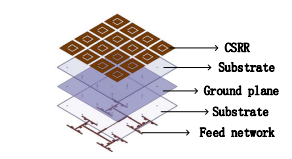## 0.3.png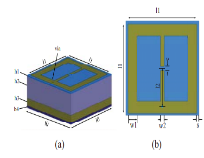## 0.4.png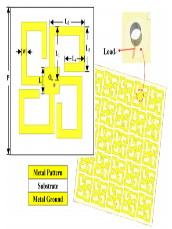## 0.5.png## 0.6.jpg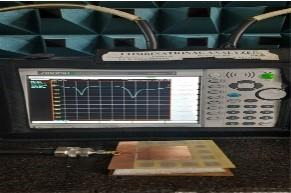Dimensions

60x60x1mm &

96mmx84mmx1mm

74cmx74cmx1mm

100x70x60mm

70mmx70mmx1mm

-

96mmx84mmx1mm

Substrates

FR4(4.4)

Rogers F4B (2.65)

RT/duroid 6006 (6.15)

Rogers substrate

10.2

Rogers R0310

FR4(4.4)

Operating frequency

2.4GHz

5.16GHz

2.45GHz

2.45GHz

-

2.4GHz

Gain

3.5dB

-

-

4.5dBi

8.0dBi

Efficiency

-

--

Antenna eff

>85.65%

Achieved maximum of 62% harvesting efficiencies at four bands

-

93.5%

Front to Back ratio

-

-

-

113.5

Output voltage

1.98Volts

-

2.82volts

Any other results

-

35.1% Efficiency achieved at

-10dB input power

Efficiency was achieved for 2mm and 6mm air gap

-

Achieved high efficiency with 113.5 of front to back ratio

7. Conclusion

The primary goal of this study is to design a metasurface antenna for use in RF energy harvesting applications in order to improve gain and bandwidth. It has been observed that from the open literature that a maximum gain of 3.5dBi and a bandwidth of 110MHz is achieved with a low output voltage.

The proposed antenna can be built with a dual substrate and a 10mm air gap, with FR4 as the dielectric material. On both substrates, the suggested antenna can be built with the metasurface plane. It was observed that the suggested antenna increased Gain 5dBi, i.e., from 3.5dBi to 8dBi, without effecting other parameters. The proposed antenna has been shown to exhibit low backward radiation, i.e., -13.1dB. The proposed antenna has fabricated using the FR4 substrates and measured using the network analyser. The entire design has done on the HFSS 19.2 and ADS 2020 software.

References

 Shire, A.M., Abdullah, N., Mohd, E. (2016). Design of rectenna for wireless energy harvesting. 2016 IEEE Student Conference on Research and Development (SCOReD), pp. 1-4. https://doi.org/10.1109/SCORED.2016.7810060

 Deepa, S.N., Rani, B.S.S. (2017). RF energy harvesting using 900MHz of mobile signal frequency to charging the mobile battery. 2017 International Conference on Innovations in Green Energy and Healthcare Technologies (IGEHT), pp. 1-5. https://doi.org/10.1109/IGEHT.2017.8094066

 Park, I. (2018). Application of metasurfaces in the design of performance-enhanced low-profile antennas. EPJ Applied Metamaterials, 5: 11. https://doi.org/10.1051/epjam/2018008

 Wagih, M., Weddell, A.S., Beeby, S. (2020). Millimeter-wave power harvesting: A review. IEEE Open Journal of Antennas and Propagation, 1: 560-578. https://doi.org/10.1109/OJAP.2020.3028220

 Saeed, W., Shoaib, N., Cheema, H.M., Khan, M.U. (2018). RF energy harvesting for ubiquitous, zero power wireless sensors. International Journal of Antennas and Propagation, 2018: 1-16. https://doi.org/10.1155/2018/8903139

 Liu, R., Wang, X., Nie, D., Wang, L., Cui, W., Wang, M., Zheng, H., Li, E. (2020). Metasurface: Enhancing gain of antenna and energy harvesting system design. International Journal of RF and Microwave Computer-Aided Engineering, 30(2). https://doi.org/10.1002/mmce.22053

 Zhao, Q., Li, L., Zhang, X., Zhang, X., Chen, J. (2018). An ambient energy harvester using metasurface. 2018 IEEE International Symposium on Electromagnetic Compatibility and 2018 IEEE Asia-Pacific Symposium on Electromagnetic Compatibility (EMC/APEMC), pp. 111-112. https://doi.org/10.1109/ISEMC.2018.8394077

 Behera, B.R., Meher, P.R., Mishra, and S.K. (2021). Metasurface superstrate inspired printed monopole antenna for RF energy harvesting application. Progress In Electromagnetics Research C, 110: 119-133. https://doi.org/10.2528/PIERC21011405

 El Badawe, M., Almoneef, T.S., Ramahi, O.M. (2017). A metasurface for conversion of electromagnetic radiation to DC. AIP Advances, 7(3): 035112. https://doi.org/10.1063/1.4978321

 Aldhaeebi, M.A., Almoneef, T.S. (2020). Planar dual polarized metasurface array for microwave energy harvesting. Electronics, 9(12): 1985. https://doi.org/10.3390/electronics9121985

 Hu, W., Inserra, D., Huang, Y., Li, J., Wen, G., Xie, J., Zhu, W. (2018). High efficiency electromagnetic energy harvesting with metasurface. 2018 IEEE Asia-Pacific Conference on Antennas and Propagation (APCAP), pp. 488-489. https://doi.org/10.1109/APCAP.2018.8538030

 Zhang, C., Zhou, Y.J., Xiao, Q.X., Yang, L., Pan, T.Y., Ma, H.F. (2016). High-efficiency electromagnetic wave conversion metasurfaces for wireless energy harvesting. 2016 Progress in Electromagnetic Research Symposium (PIERS), pp. 1720-1722. https://doi.org/10.1109/PIERS.2016.7734772

 Ghaderi, B., Nayyeri, V., Soleimani, M., Ramahi, O.M. (2017). A novel symmetric ELC resonator for polarization-independent and highly efficient electromagnetic energy harvesting. 2017 IEEE MTT-S International Microwave Workshop Series on Advanced Materials and Processes for RF and THz Applications (IMWS-AMP), pp. 1-3. https://doi.org/10.1109/IMWS-AMP.2017.8247443

 Faenzi, M., Minatti, G., González-Ovejero, D., Caminita, F., Martini, E., Della Giovampaola, C., Maci, S. (2019). Metasurface antennas: New models, applications and realizations. Scientific Reports, 9(1): 10178. https://doi.org/10.1038/s41598-019-46522-z

 Abdulkarim, Y.I., Awl, H.N., Muhammadsharif, F.F., Karaaslan, M., Mahmud, R.H., Hasan, S.O., Işık, Ö., Luo, H., Huang, S. (2020). A low-profile antenna based on single-layer metasurface for Ku-band applications. International Journal of Antennas and Propagation, 2020: e8813951. https://doi.org/10.1155/2020/8813951

 Alsager, A.F. (2011). Design and analysis of microstrip patch antenna arrays. Electrical Engineering–Communication and Signal Processing, College of Borås. https://www.divaportal.org/smash/get/diva2:1312395/FULLTEXT01.pdf.